Next: Two-Particle Systems Up: Multi-Particle Systems Previous: Fundamental Concepts

# Non-Interacting Particles

In general, we expect the Hamiltonian of a multi-particle system to take the form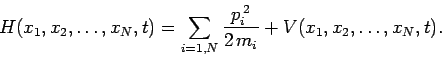(426)

Here, the first term on the right-hand side represents the total kinetic energy of the system, whereas the potentialspecifies the nature of the interaction between the various particles making up the system, as well as the interaction of the particles with any external forces.

Suppose that the particles do not interact with one another. This implies that each particle moves in a common potential: i.e.,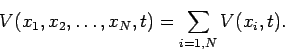(427)

Hence, we can write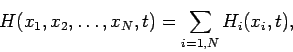(428)

where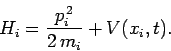(429)

In other words, for the case of non-interacting particles, the multi-particle Hamiltonian of the system can be written as the sum ofindependent single-particle Hamiltonians. Here,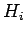represents the energy of theth particle, and is completely unaffected by the energies of the other particles. Furthermore, given that the various particles which make up the system are non-interacting, we expect their instantaneous positions to be completely uncorrelated with one another. This immediately implies that the multi-particle wavefunction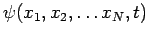can be written as the product ofindependent single-particle wavefunctions: i.e.,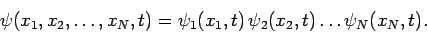(430)

Here,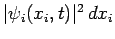is the probability of finding theth particle between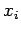and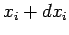at time. This probability is completely unaffected by the positions of the other particles. It is evident that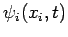must satisfy the normalization constraint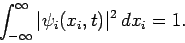(431)

If this is the case then the normalization constraint (418) for the multi-particle wavefunction is automatically satisfied. Equation (430) illustrates an important point in quantum mechanics: namely, that we can generally write the total wavefunction of a many degree of freedom system as a product of different wavefunctions corresponding to each degree of freedom.

According to Eqs. (428) and (430), the time-dependent Schrödinger equation (423) for a system ofnon-interacting particles factorizes intoindependent equations of the form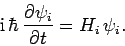(432)

Assuming that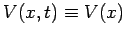, the time-independent Schrödinger equation (425) also factorizes to give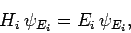(433)

where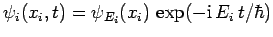, and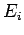is the energy of theth particle. Hence, a multi-particle state of definite energyhas a wavefunction of the form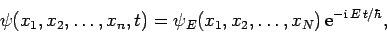(434)

where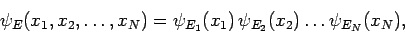(435)

and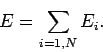(436)

Clearly, for the case of non-interacting particles, the energy of the whole system is simply the sum of the energies of the component particles.Next: Two-Particle Systems Up: Multi-Particle Systems Previous: Fundamental Concepts
Richard Fitzpatrick 2010-07-20